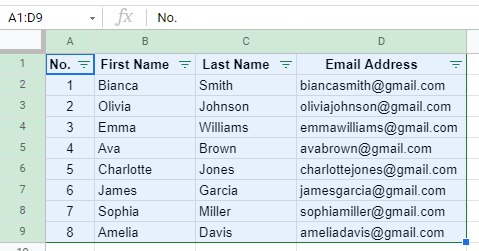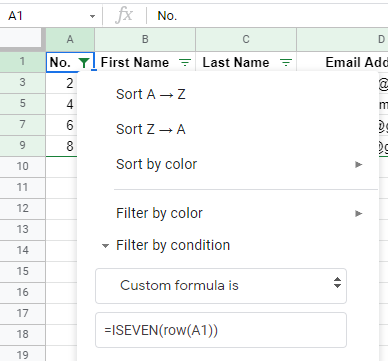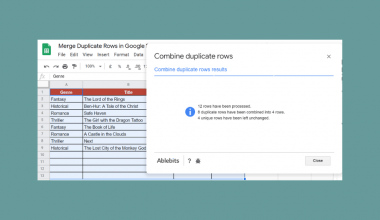# How to Delete Every Other Row in Google Sheets

By learning how to delete every other row in Google Sheets is useful when you want to delete rows quickly and not manually or manipulate the data by deleting certain rows

This tutorial is to teach you to delete and hide every other row in Google Sheets by using the filter tool.

The filter tool can not only assist us to deleting every other row, but it can also use advanced formulas to delete every third, fourth, or Nth row in a dataset.

Let’s start! 🤗

## Deleting Every Other Row in Google Sheets Using the Filter Tool

It is really simple to delete every other row in Google Sheets. We would simply use the filter tool to hide either the even or odd-numbered rows.

Let’s use an example to show you what we mean!

#### Example 1:

1. First, we would need to select the headers we would like to filter. In our example, it would be A1:D1.1. Find the filter tool. It would be on the top right side of your toolbar. Once you have found it, click it.1. After clicking on the filter tool, your headers would appear several filter icons.1. Then you click on the filter icon, a drop-down would appear. We would then click on ‘Filter by condition. It would then allow you to select several options. We would scroll all the way down to select ‘Custom formula is to insert a customized formula as a filter.1. The formula we are going to use would help us to hide all even-numbered rows. We will insert `=ISEVEN(row(A1))` into the box. Then press Enter.1. After pressing Enter, your input should look like this.As you can see, the filter has to hide every other row that is even-numbered. Another thing to take note of is that the formula we input in filters the rows that are even-numbered instead of the data that are even-numbered.

Here is a breakdown of the formula we used in the formula.

1. `ISEVEN` is a function; it is used to check the presence of even integer values in cells.
2. `ROW` is a command. So instead of filtering a single cell, it would filter the whole row.

So together, the formula will help us to hide all even-numbered rows.

## Deleting Every Nth Row in Google Sheets Using the Filter Tool

Other than deleting or hiding every other row in Google Sheet, you can also use another formula in the filter tool to delete or hide every third, fourth or fifth row.

This formula is a bit more complex than the previous formula. However, it is also very customizable.

Let’s use the same database as Example 1 to show how this can be achieved.

#### Example 2:

So instead of inserting the previous formula into the filter tool, we would be using the MOD function.

1. We would like to hide every third row excluding the header. The image below shows that every third row excluding the header would be highlighted in green.1. To do so, we will enter `=MOD((row(A1) - row (\$A\$1)-2),3)` into the filter.1. The filter would then filter out every third row:You can see that the highlighted rows are now missing, meaning the formula inputted in the filter tool works!

Let’s have a better understanding of what the formula does.

1. The `MOD` function is being used as a division operator, where if the return is a remainder of “0”, we would hide the cell.
2. ROW is a command. So instead of filtering a single cell, it would filter the whole row.
3. The “-2” means that the filter would hide the third row after the header.
4. The “3” means the numeric value that sets the row to hide interval. Since we want to hide every third row, hence we input ‘3.

You may make a copy of the spreadsheet using the link attached below and try it for yourself:

As simple as that! You can now hide or delete every other row in Google Sheet by following the steps above.

Don’t be afraid to play with the formulas. With little modification, you can also customize the formula in the way you prefer!Our goal this year is to create lots of rich, bite-sized tutorials for Google Sheets users like you. If you liked this one, you'll love what we are working on! Readers receive ✨ early access ✨ to new content.

##### You May Also Like## How to Make a Bubble Chart in Google Sheets

Making a bubble chart in Google Sheets is useful for depicting three or four dimensions of data. At…## How to Use IMARGUMENT Function in Google Sheets

You can use the IMARGUMENT function in Google Sheets when you need to know the angle or theta…## How to Merge Duplicate Rows in Google Sheets

To merge duplicate rows in Google Sheets seems complicated to me. There are times when I don’t really…## How to Set Print Area in Google Sheets

Setting a print area lets you define the section in your spreadsheet that will be included in the…